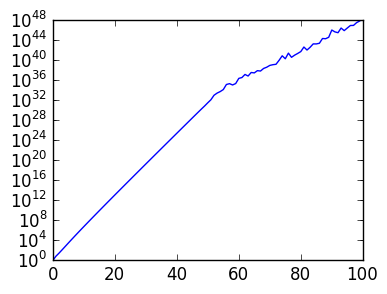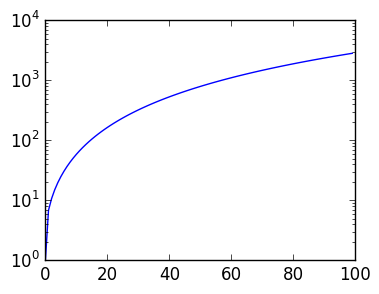# Assignment 2¶

This lab explores two alternative decompositions to the LU and QR decompositions: the UL Decomposition and LQ decompositions.

## UL Decomposition¶

An LU decomposition is constructed by multiplying by a lower triangular matrix that introduces zeros column-by-column starting with the first column.

Instead, we will investigate the UL decomposition: we will introduce zeros column-by-column starting with the last column.

Exercise 1(a) Given an $n \times n$ matrix A, what upper triangular matrix U_j introduces zeros in the $j$th column:

(U_j*A)[1:j-1,j] == zeros(1:j-1)?


Complete the following function that takes in a square Matrix A and an integer j and returns an upper triangular Matrix U_j that satisfies the above property.

In :
# INPUT:  A square matrix A and integer j
# OUTPUT: A matrix U_j that is upper triangular

function upper_reduce_col(A,j)
n=size(A,1)
U=eye(n)

for k=1:j-1
U[k,j]=-A[k,j]/A[j,j]
end

U
end

A=rand(5,5)
U=upper_reduce_col(A,4)
U*A

Out:
5x5 Array{Float64,2}:
0.079882  -0.0327976  -0.312991   1.11022e-16  -0.0893706
0.817018   0.235419    0.0803426  0.0           0.508066
0.165188   0.340396    0.713421   0.0           0.326151
0.456192   0.702257    0.656478   0.892522      0.481106
0.843693   0.24602     0.341197   0.703776      0.0503664

Exercise 1(c) Check that

n=100
A=rand(n,n)
U_n=upper_reduce_col(A,n)
norm((U_n*A)[1:n-1,n])


is small.

In :
n=100
A=rand(n,n)
U_n=upper_reduce_col(A,n)
norm((U_n*A)[1:n-1,n])

Out:
3.764949453935611e-16

Exercise 1(d) Complete the following function that returns a UL Decomposition.

In :
# INPUT:  A square matrix A
# OUTPUT: A tuple (U,L) where U is upper triangular and L is lower triangular

function ul(A)
n=size(A,1)

Ui=eye(n)
for j=n:-1:2
Uj=upper_reduce_col(A,j)
Ui=Uj*Ui
A=Uj*A
end

inv(Ui),A
end

Out:
ul (generic function with 1 method)

Exercise 1(e) Check that the following is small:

In :
n=100
A=rand(n,n)
U,L=ul(A)

norm(U*L-A)

Out:
4.935716917863025e-10

Recall from lectures the matrix that breaks the PLU Decomposition

$$A_n \triangleq \begin{pmatrix} 1 & && & 1 \cr -1 & 1 && & 1\cr -1 & -1 & 1 && 1 \cr \vdots & \vdots & \vdots & \ddots & \vdots \cr -1 & -1 & -1 & \cdots & 1 \end{pmatrix}$$

Exercise 2(a) Write a routine bad_plu_mat(n) that takes in an integer n and returns an n x n Matrix $A_n$ as defined above. Use a different approach to construct the matrix than what was done in lectures.

In :
# INPUT:  An Integer n
# OUTPUT: A Matrix A_n that is n x n

A=2eye(n)-tril(ones(n,n))
A[1:n-1,end]=ones(n-1)
A
end


Out:
(
3x3 Array{Float64,2}:
1.0   0.0  1.0
-1.0   1.0  1.0
-1.0  -1.0  1.0,

4x4 Array{Float64,2}:
1.0   0.0   0.0  1.0
-1.0   1.0   0.0  1.0
-1.0  -1.0   1.0  1.0
-1.0  -1.0  -1.0  1.0)

Exercise 2(b) Plot the condition numbers ${\rm cond}(L)*{\rm cond}(U)$ for $A_n = P L U$ as a function of n, for n=1:100, using semilogy.

In :
using PyPlot

(L,U,p)=lu(A);
cond(L)*cond(U)) for n=1:100]

semilogy(cnd)Out:
1-element Array{Any,1}:
PyObject <matplotlib.lines.Line2D object at 0x31329bf10>

Exercise 2(c) Plot the condition numbers ${\rm cond}(U)*{\rm cond}(L)$ for $A_n = UL$ as a function of n, for n=1:100, using semilogy. What do you conclude about the stability of the UL decomposition for this example?

In :
cnd=Float64[(A=bad_plu_mat(n);
(U,L)=ul(A);
cond(U)*cond(L)) for n=1:100]

semilogy(cnd);# LQ Decomposition¶

This part investigates the calculation of $A=LQ$, which is an alternative to $A=QR$.

Exercise 2(a) Write a function house(x,j) that takes in a Vector x and returns an orthogonal Matrix $Q$ so that

$$x^\top Q = [x_1,\ldots,x_{j-1},-{\rm sign}\, x_j \|x_{j:end}\|,0,\ldots,0]$$
In :
# INPUT:  A Vector x and Integer j
# OUTPUT: A Matrix Q that is m x m and orthogonal

function house(x,j)
# TODO: Return a Householder reflection Q so that
#    QA=Q*A satsifies QA[j+1:n,j]==zeros(n-j) and QA[1:j-1,j]==A[1:j-1,j]
# for     n=size(A,1)
n=length(x)
x=[zeros(j-1);x[j:end]]
α=-sign(x[j])
v=x-α*norm(x)*[zeros(j-1);1;zeros(n-j)]
v=v/norm(v)
I-2v*v'
end

x=rand(5)
Q=house(x,2)
x'*Q

Out:
1x5 Array{Float64,2}:
0.906057  -1.39361  -5.55112e-17  -1.73472e-16  -2.22045e-16

Exercise 2(b) Check numerically that

$$\|x^\top Q - [x_1,\ldots,x_{j-1},-{\rm sign}\, x_j \|x_{j:end}\|,0,\ldots,0]\|$$

is small for a random vector of length 100 and j=3.

In :
x=rand(100)
Q=house(x,3)
norm(Q*x-[x;x;-sign(x)*norm(x[3:end]);zeros(length(x)-3)])

Out:
2.479007607181183e-15

Exercise 2(c) Use part 2(a) to write a function lq(A) that calculates the RQ Decomposition: it takes in a n x m Matrix A and returns a tuple of matrices L and Q so that L is n x m and lower triangular, Q is m x m and orthogonal, and L*Q is (up to rounding error) equal to A.

In :
# INPUT:  An n x m matrix A
# OUTPUT: A tuple (L,Q)  where L is n x m lower triangular and Q is m x m and orthogonal

function lq(A)
n,m=size(A)
Qt=eye(m)
for k=1:min(n,m)
Q=house(A[k,:],k)
A=A*Q
Qt=Qt*Q
end

A,Qt'
end

A=rand(5,4)
L,Q=lq(A)
norm(L*Q-A),norm(Q'*Q-I)

A=rand(4,5)
L,Q=lq(A)
norm(L*Q-A),norm(Q'*Q-I)

A=rand(4,4)
L,Q=lq(A)
norm(L*Q-A),norm(Q'*Q-I)

Out:
(2.5104854386371074e-15,1.677820222479436e-15)

Exercise 2(d) Demonstrate numerically that your lq routine satisfies $\|A-LQ\|$ is small for random matrices for the cases $n > m$, $n=m$ and $n < m$.

In :
A=rand(10,5)
L,Q=lq(A)
norm(A-L*Q)

Out:
1.2216993950229764e-15
In :
A=rand(5,10)
L,Q=lq(A)
norm(A-L*Q)

Out:
8.420114109796008e-16
In :
A=rand(10,10)
L,Q=lq(A)
norm(A-L*Q)

Out:
2.7239005432278633e-15
In [ ]: